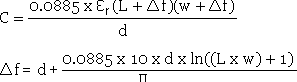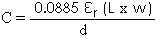# Capacitance of a rectangular capacitor, with edge effect, calculator

This calculator can be used for finding the capacitance between PCB layers. It uses a formula derived at Chemandy Electronics which is unconventional but produces very useful results. Use it at your own risk and see the notes below.

 INPUT DATA Relative Dielectric Constant (εr): Width: cm Length: cm Dielectric Thickness: mm
 RESULTS Capacitance without edge effect: pF Capacitance including edge effect: pF

This calculator uses JavaScript and will function in most modern browsers. For more information see About our calculators

After a long unsuccessful search for a suitable accurate formula, I derived the formula above mainly for use when designing PCB,s and measuring Er. It assumes that there is a mirror ground plane on the bottom of the board and dielectric extending outside the edges of the (copper) plates. I have found the formula to be reasonably accurate on FR4 and it 'tracks' well to Kirchhoff's formula for a circular capacitor with an equivalent area, thickness and Er. It also appears to work fairly well on PCB tracks (using Eeff). I make no claim that this formula will work for anything other than a PCB and reserve the right to change it as and when more valid data is available to me. Hopefully this calculator will stimulate some discussion which could lead to a more accurate formula. It has been pointed out that it is not possible to take the log of L*w by two separate people (because it is a dimensioned number) but as yet no solution has been offered for this. Please use with caution and give us some feedback, good or bad.

The capacitance of a rectangular or square parallel plate capacitor including edge effect, can be calculated using the following formula.Where:

L is the Length in cm

w is the Width in cm

εr is the Relative Dielectric Constant

d is the Dielectric thickness in cm

The capacitance is also given without edge effect for comparison purposes and uses the formula.This calculator is provided free by Chemandy Electronics in order to promote the FLEXI-BOX